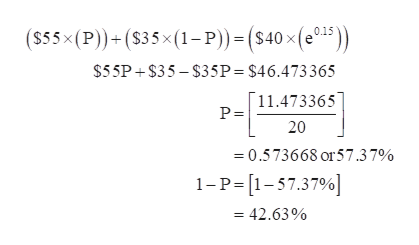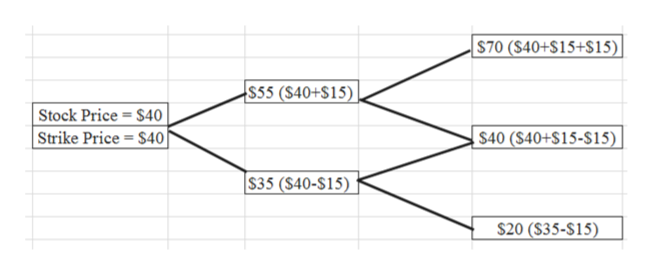# The current price of XL Corporation stock is \$40. In each of the next two years, this stock price can either go up by \$15.00 or go down by \$15.00.  XL stock pays no dividends.  The one-year risk-free interest rate is 15% and will remain constant. Using the binomial pricing model, calculate the price of a two-year American put option on LC stock with a strike price of \$40.

Question
51 views

The current price of XL Corporation stock is \$40. In each of the next two years, this stock price can either go up by \$15.00 or go down by \$15.00.  XL stock pays no dividends.  The one-year risk-free interest rate is 15% and will remain constant.

Using the binomial pricing model, calculate the price of a two-year American put option on LC stock with a strike price of \$40.

check_circle

Step 1

Calculate the probabilities:

p is 57.37% and 1-p is 42.63%.

Step 2help_outlineImage Transcriptionclose(S55x (P))+ (s35x(1- P) = (S40 x (e3 0.15 \$55P+\$35-\$35P = \$46.473365 11.473365 P= 20 0.573668 or57.37% 1-P-[1-57.37% =42.63% fullscreen
Step 3

Create a binomial model:

...help_outlineImage Transcriptionclose\$70 (\$40+S15+\$15) \$55 (\$40+\$15) Stock Price = \$40 Strike Price = \$40 \$40 (\$40+S15-\$15) \$35 (\$40-\$15) \$20 (\$35-\$15) fullscreen

### Want to see the full answer?

See Solution

#### Want to see this answer and more?

Solutions are written by subject experts who are available 24/7. Questions are typically answered within 1 hour.*

See Solution
*Response times may vary by subject and question.
Tagged in